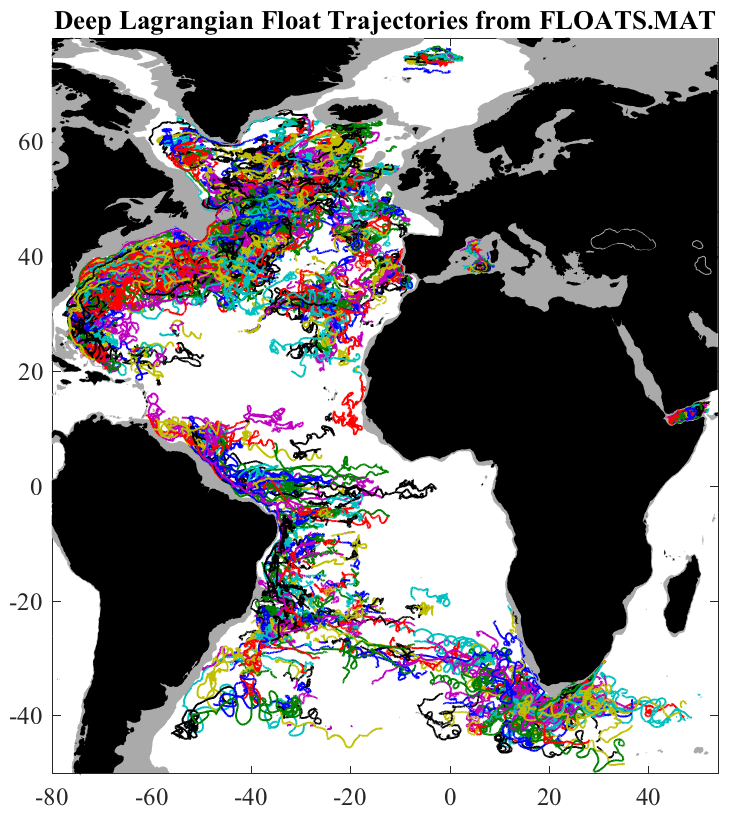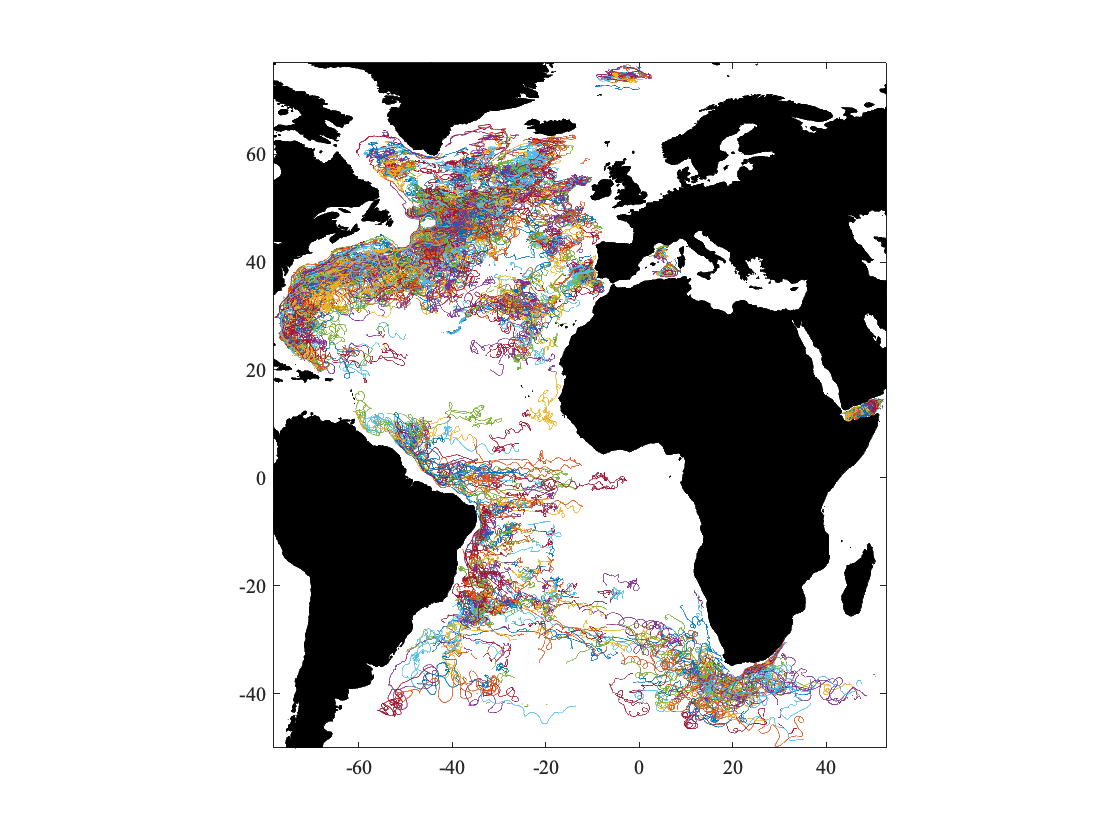# Distributional Data Analysis

In this lab we will look at a way of working with data that I call "Distributional Data Analysis". This is a simple but extremely powerful method that is just as suitable for a single time series as it is for a giant dataset of tens of millions of data points. This approach is my starting place for looking at pretty much anything. The meaning of the name will become clear as we proceed through the lab.
To begin with we need a data set to work with. I would like you to get the hang of working with large, messy data sets. Therefore we are going to work with a data set that I've put together called floats.mat. This contains more or less the trajectory of every freely drifting deep Lagrangian float ever deployed.These experiments took place over many decades and represent an enormous amount of time and resources in their creation and processing. It's a pretty amazing dataset to work with!

A Lagragian float is an instrument that is designed to be the same density as seawater at a prescribed depth, usually between 200 and 1200 m but sometmes much deeper. The word "Lagrangian" means that the instrument is intended to follow the fluid motion, a reference to a Lagrangian or particle-following coordinate system, as opposed to an Eulerian or fixed coordinate system.
Such floats are called RAFOS floats. To understand this name, we need to have a small history lesson. In the early days the floats themselves were sounds sources, and their positions were determined by triangulating acoustic travel times between the floats and a set of fixed underwater receivers. These were called SOFAR floats for SOund Fixing And Ranging. However, the floats had to be pretty big in order to power the sound sources.
Later, it was realized that it made a lot more sense if the roles of source and receiver were reversed. The floats became receivers, and the fixed moorings became the sound sources. This reversed system was given the name RAFOS by reversing the SOFAR acroymn. These floats, which were introduced by Tom Rossby and colleagues in 1986, remain one of the most important platforms for in situ observations of the ocean currents.
Floats.mat collects together all historical publicly-available RAFOS float trajectories, as well as some SOFAR trajectories from the early days. Please visit my website and download http://www.jmlilly.net/ftp/pub/floats.zip, then follow the directions in the "readme" file therein.

# Getting Started with Floats.mat

Now you should be able to evaluate the following command:
floats
floats = struct with fields:
experiments: {33×1 cell}
expid: [1469×1 double]
id: {89×1 cell}
dt: [1469×1 double]
num: {1469×1 cell}
lat: {1469×1 cell}
lon: {1469×1 cell}
p: {1469×1 cell}
t: {1469×1 cell}
cv: {1469×1 cell}
If that didn't work it means the steps in the readme were probably not followed correctly. If you want explanations about the variable names, you can type "help about_floats".
The first thing you notice is that most of the variables are things called "cells". A cell array is a very useful type of data structure in Matlab, so it's worthwhile to take a few minutes to introduce it to you.

# Introducing Cell Arrays

The floats.mat dataset consists of primarily of the positions of floats as they follow the currents, giving us time series of latitude and longitude. However, all the floats trajectories are of different lengths.
Let's say that we were to try to store this data as a matrix. We could put time in rows, with each column corresponding to a different float. By the way, this is a good time to mention that in my convention, time is always oriented to increase in the row direction. In other words, if I work with a single time series, I always use a column vector, and not a row vector. In Matlab x=[1:10]' is a column vector while x=[1:10] is a row vector.
If we were to store the float data as a matrix, then the number of rows would have to be the same as the number of data points of the longest trajectory. This would be very inefficient because we would then have a lot of empty space in the matrix. It works ok for small datasets, but for large data sets you can quickly run into memory trouble. So it doesn't really make sense to store this data as a matrix.
A cell array offers us an easy solution. A cell array is like a regular array, except the entries can be anything. Here is an example:
a{1}='apple';
a{2}='banana';
a{3}='pear';
a
a = 1×3 cell array
{'apple'} {'banana'} {'pear'}
Individual entries of cell arrays are accessed through curly braces ...
a{2}
ans = 'banana'
...whereas parantheses let us access a subset of the cell array itself:
a(1:2)
ans = 1×2 cell array
{'apple'} {'banana'}
Note that a(1:2) is another cell array, where as a{1} is a string in this case.
Cell arrays can also be of different sizes, and can even combine objects of different types:
b{1,1}='age';
b{1,2}=46;
b{2,1}='height';
b{2,2}=179;
b
b = 2×2 cell array
{'age' } {[ 46]}
{'height'} {}
Now b{1,1} is a string while b{1,2} is an integer. As you can see cell arrays are pretty flexible. However, in what follows we are going to limit ourselves to working with one particular format, cell arrays of numerical arrays.
To keep track of arrays of different lengths, we can store them as a cell array.
x=[1:10]'; %the prime in Matlab is the transpose
y=[1:20]'; %(or conjugate transpose for complex valued-arrays)
z=[1:4]'; %it converts a row vector into a column
c{1,1}=x;
c{2,1}=y;
c{3,1}=z;
c
c = 3×1 cell array
{10×1 double}
{20×1 double}
{ 4×1 double}
Now c is a cell array in the shape of a column vector, and each of its elements is itself a column vector. We can think of this as being like stacking x, y, and z on top of each other. (Note that if we had just written c{1}=x rather than c{1,1}=x and so forth, c would have become a row vector than a column vector.)
For simplicity we will refer to this format as "cell array format." This is the format used by floats.mat. This turns out to be hugely useful in many applications. I use it so often I wrote a whole module for working with data in this format called jCell, which you can read about by typing "help jcell".
For now though, I just wanted to explain cell arrays and the basic structure of the floats.mat variables.

# Plotting the Dataset

After that detour into cell arrays, we're now ready to plot the data. A handy jCell function called cellplot lets us plot data in cell array format without needing to loop. Go ahead and evaluate the following, which might take a while because the data is pretty large:
use floats
cellplot(lon,lat),axis tight
topoplot continents
latratio(30)Here topoplot is a convenient function for plotting bathymetry and topography. We will look at this more later so you don't need to worry about it too much for now. The other command, latratio, sets the aspect ratio of the plot correct for the input latitude. In other words, latratio(30) sets the aspect ratio to be 1 to 1 at 30 degrees latitude. This enables us to decide what aspect ratio we want a latitude/longitude plot to be. Otherwise, stretching a figure window in Matlab will stretch latitude and longitude accordingly, and we can often end up with some really distorted looking maps.
You can get a better view of this dataset by mousing over the figure and looking for a small arrow in the upper right-hand corner. Clicking on this will open the figure in its own window.

# Two Dimensional Histograms

After all of that we are finally ready to begin working with the distributional data analysis. To make things a little easier, we are going to focus on the North Atlantic portion of this data set. Firstly we want to examine the data density, that is, the number of observations per grid point. To do this we will make a kind of plot called a two-dimensional histogram.
A two-dimensional histogram simply counts the number of data points within each bin of a two-dimensional grid. These are made in jLab by the routine twodhist. Matlab's histcounts2 provides the same functionality.
Go ahead and evaluate the code below.
[mat,xmid,ymid]=twodhist(lon,lat,[-80:1/2:0],[15:1/2:65]);
clf,jpcolor(xmid,ymid,log10(mat))
caxis([0.5 2.5]),colorbar
topoplot continents